2016-06-20 11:21:24 sungo2012 阅读数 184
• ###### 区块链技术详解

区块链技术入门教程，该课程对目前区块链知识进行一次体系化的讲解，使得初学者能迅速抓住区块链技术的核心思想，对区块链应用和智能合约有一个的、由浅入深的了解。

12379 人正在学习 去看看 CSDN讲师

# 1、前言

1、Visual C++ 数字图像模式识别典型案例详解

2、Visual C++数字图像处理技术详解

2019-08-22 09:57:02 weixin_42618420 阅读数 412
• ###### 区块链技术详解

区块链技术入门教程，该课程对目前区块链知识进行一次体系化的讲解，使得初学者能迅速抓住区块链技术的核心思想，对区块链应用和智能合约有一个的、由浅入深的了解。

12379 人正在学习 去看看 CSDN讲师

# 图像分割技术

## 边缘分割技术

``````I = imread('gantrycrane.png');
I = rgb2gray(I);
h1 = [-1 -1 -1; 2 2 2; -1 -1 -1]; %水平
h2 = [-1 -1 2; -1 2 -1; 2 -1 -1]; %+45度
h3 = [-1 2 -1; -1 2 -1; -1 2 -1]; %竖直
h4 = [2 -1 -1; -1 2 -1; -1 -1 2]; %-45度

J1 = imfilter(I, h1); %线段检测
J2 = imfilter(I, h2);
J3 = imfilter(I, h3);
J4 = imfilter(I, h4);

J = J1 + J2 + J3 + J4; %四条线段叠加
figure;
subplot(121),imshow(I);
subplot(122),imshow(J);``````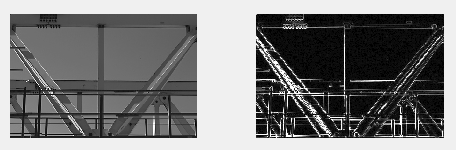## 微分算子

### Roberts算子

``````I = imread('rice.png');
I = im2double(I);
[J, thresh] = edge(I, 'Roberts', 35/255); %边缘检测 ,roberts算子, 阈值为归一化后的35/255
figure;
subplot(121),imshow(I);
subplot(122),imshow(J);``````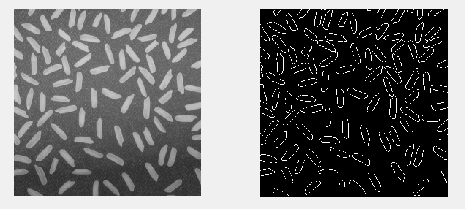### Prewitt 算子

``````I = imread('cameraman.tif');
I = im2double(I);
[J, thresh] = edge(I, 'prewitt', [], 'both');%默认阈值, 从水平和竖直方向进行设置
figure;
subplot(121);imshow(I);
subplot(122);imshow(J);
``````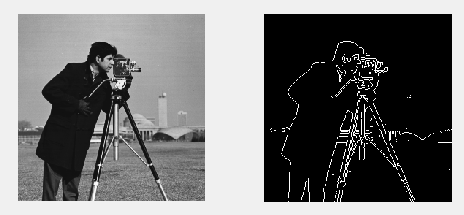### Sobel 算子

``````I = imread('gantrycrane.png'); %RGB彩色图
% imshow(I);
I = rgb2gray(I);
[J, thresh] = edge(I, 'sobel', [], 'horizontal');
figure;
subplot(121);imshow(I);
subplot(122);imshow(J);``````### Canny 算子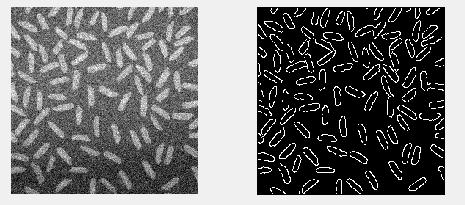### LOG 算子

``````I = imread('cameraman.tif');
I = im2double(I);
J = imnoise(I, 'gaussian', 0, 0.005);
[K, thresh] = edge(J, 'Log', [], 2.3);
figure;
subplot(121),imshow(I);
subplot(122),imshow(K);``````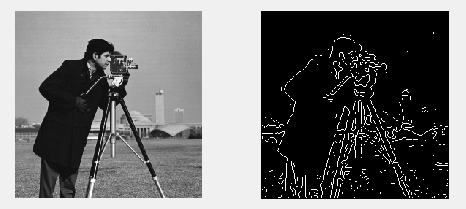## 阈值分割技术

### 全局阈值

``````I = imread('rice.png');
subplot(121),imshow(I);
subplot(122),imhist(I, 200); %直方图显示``````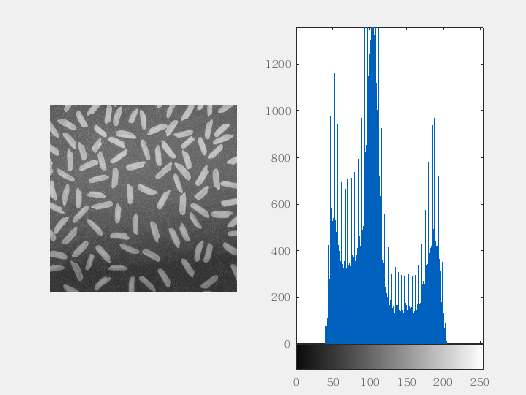### 全局阈值分割

``````I = imread('rice.png');
J = I > 120; %全局阈值为120 , 波谷大概是120
K = I > 130; %全局阈值为130
figure;
subplot(131),imshow(I);
subplot(132),imshow(J);
subplot(133),imshow(K);``````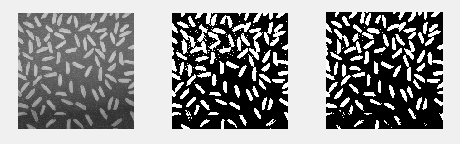### Otsu阈值分割

``````I = imread('coins.png');
I = im2double(I);
T = graythresh(I); %获取阈值
J = im2bw(I, T); %图像分割
subplot(121),imshow(I);
subplot(122),imshow(J);
``````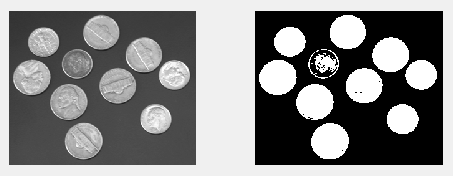### 迭代式阈值分割法

``````clear, clc
I = im2double(I);
T0 = 0.01; %精度
% T1 = graythresh(I) %用Otsu求阈值
T1 = min(I(:)) + max(I(:)) / 2; %初始估计阈值
r1 = find(I > T1); %找出比阈值大的像素
r2 = find(I <= T1); %找出比阈值小的像素
T2 = (mean(I(r1)) + mean(I(r2))) / 2; %各个像素加和求平均
while abs(T2 - T1) < T0 %
T1 = T2;
r1 = find(I > T1);
r2 = find(I <= T1);
T2 = (mean(I(r1)) + mean(I(r2))) / 2;
end

J = im2bw(I, T2);
subplot(121),imshow(I);
subplot(122),imshow(J);``````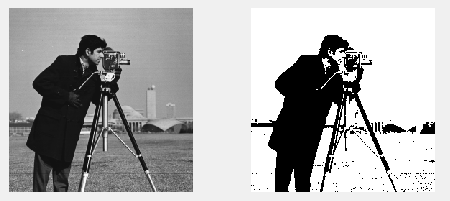## 区域分割技术

### 分水岭分割

``````clear,clc;
J = watershed(I, 4); % 4个连通区域,默认为8
subplot(121),imshow(I);
subplot(122),imshow(J);
``````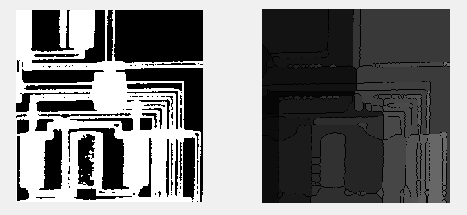2015-05-04 22:21:04 sinat_27174709 阅读数 266
• ###### 区块链技术详解

区块链技术入门教程，该课程对目前区块链知识进行一次体系化的讲解，使得初学者能迅速抓住区块链技术的核心思想，对区块链应用和智能合约有一个的、由浅入深的了解。

12379 人正在学习 去看看 CSDN讲师

《c++ Primer》第五版 中文版 看了大半，正在理解与训练中

《深入理解计算机系统》对底层研究很有帮助

《数字图像处理》第三版 学习中，为了指纹识别打下基础

《算法导论》锻炼算法能力和数学能力

《算法引论》同上，这个看着更轻松

《编程之美》翻阅

《编程珠玑》翻阅

《Visual C++ 数字图像处理技术详解》 纯技术书，练手

《Windows游戏编程之从零开始》小时候的梦想，现在看看还适不适合这条路

2018-08-16 22:54:17 Eastmount 阅读数 9743
• ###### 区块链技术详解

区块链技术入门教程，该课程对目前区块链知识进行一次体系化的讲解，使得初学者能迅速抓住区块链技术的核心思想，对区块链应用和智能合约有一个的、由浅入深的了解。

12379 人正在学习 去看看 CSDN讲师

PS：请求帮忙点个Star，哈哈，第一次使用Github，以后会分享更多代码，一起加油。

1.图像基础知识
2.OpenCV读写图像
3.OpenCV像素处理

PS: 文章也学习了网易云高登教育的知识，推荐大家学习。

PSS：2019年1~2月作者参加了CSDN2018年博客评选，希望您能投出宝贵的一票。我是59号，Eastmount，杨秀璋。投票地址：https://bss.csdn.net/m/topic/blog_star2018/index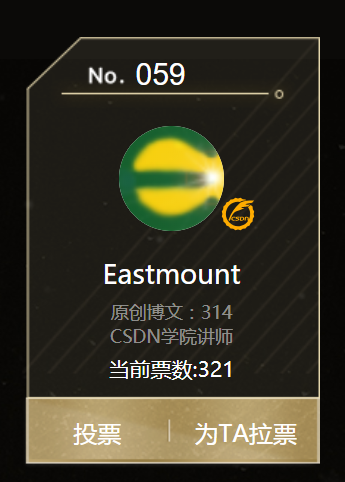# 一.图像基础知识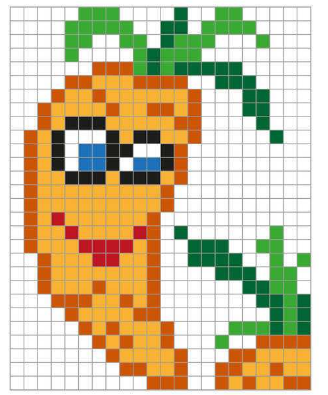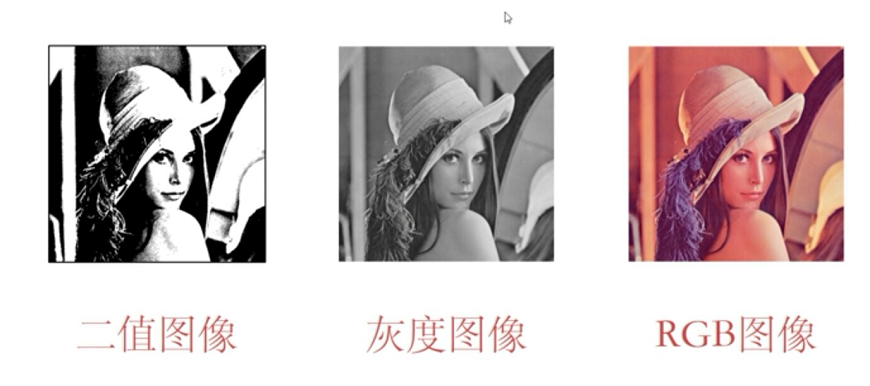1.二值图像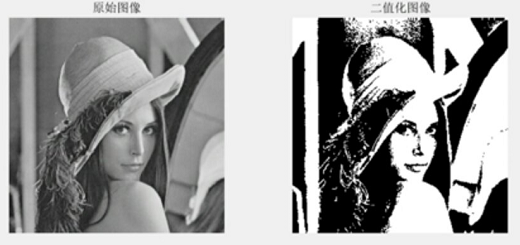2.灰度图像

(1) 浮点算法：Gray=R0.3+G0.59+B0.11
(2) 整数方法：Gray=(R
30+G59+B11)/100
(3) 移位方法：Gray=(R28+G151+B77)>>8;
(4) 平均值法：Gray=（R+G+B）/3;(此程序采用算法)
(5) 仅取绿色：Gray=G；
(6) 加权平均值算法：根据光的亮度特性，公式: R=G=B=R
0.299+G*0.587+B0.144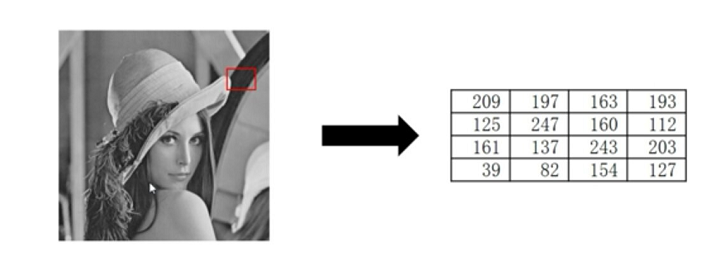3.彩色图像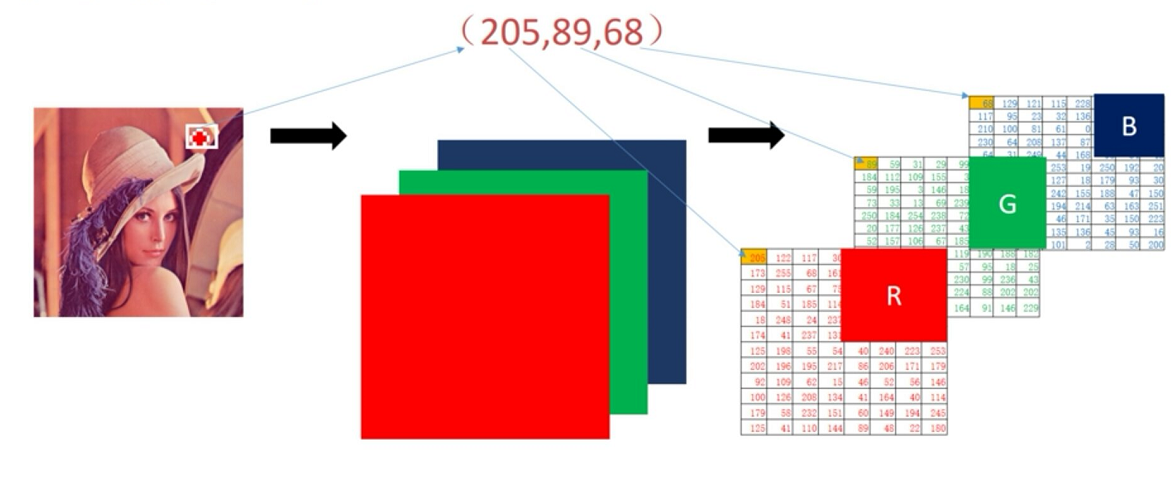# 二.OpenCV读写图像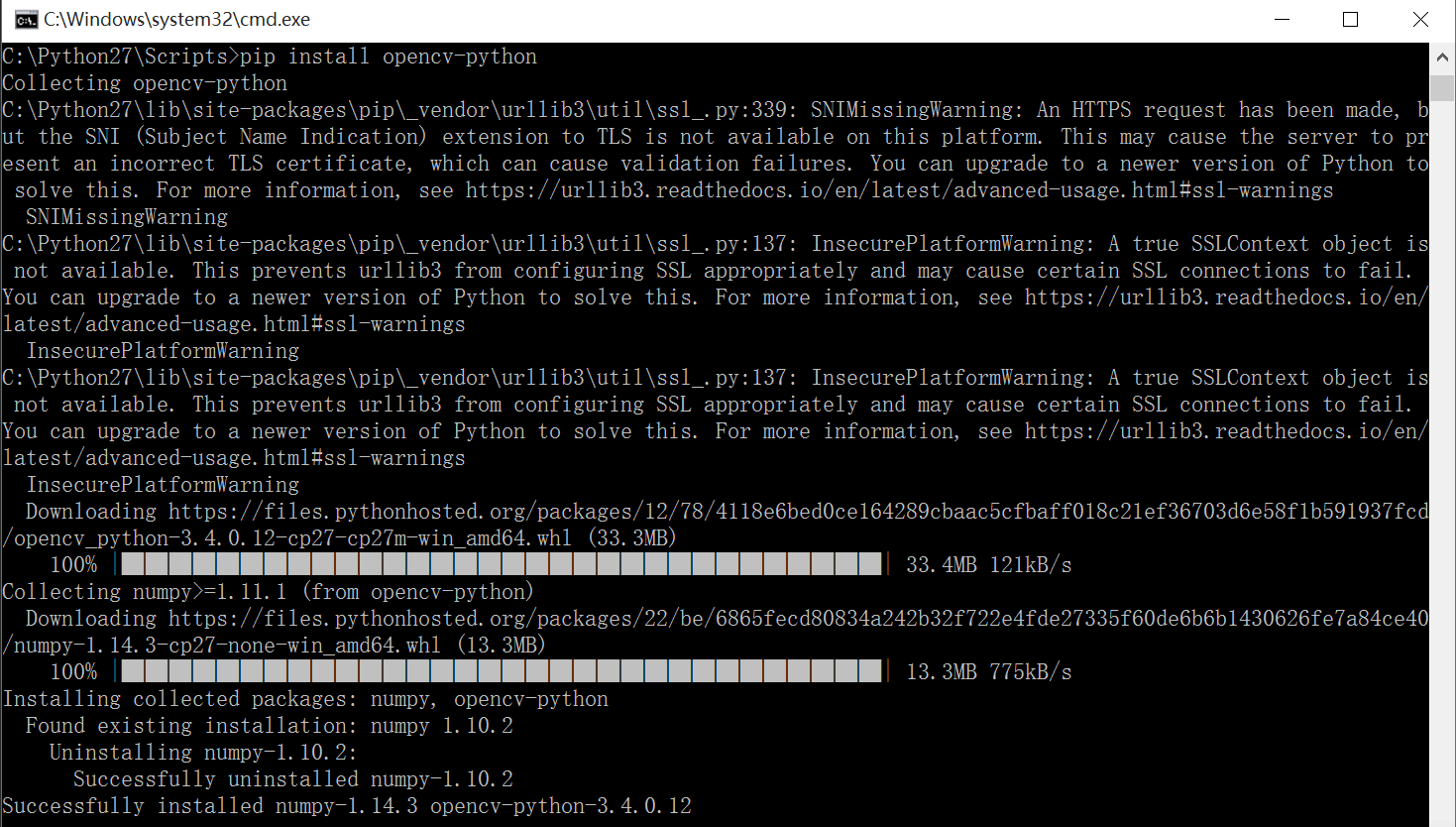1.读入图像
OpenCV读图像主要调用下面函数实现：

2.显示图像

cv2.imshow(窗口名, 图像名)

3.窗口等待

cv2.waitKey(delay)

4.删除所有窗口

cv2.destroyAllWindows() 删除所有窗口
cv2.destroyWindows() 删除指定的窗口

5.写入图片

retval = cv2.imwrite(文件地址, 文件名)

``````# -*- coding:utf-8 -*-
import cv2

#读取图片

#显示图像
cv2.imshow("Demo", img)

#等待显示
cv2.waitKey(0)
cv2.destroyAllWindows()

#写入图像
cv2.imwrite("testyxz.jpg", img)
``````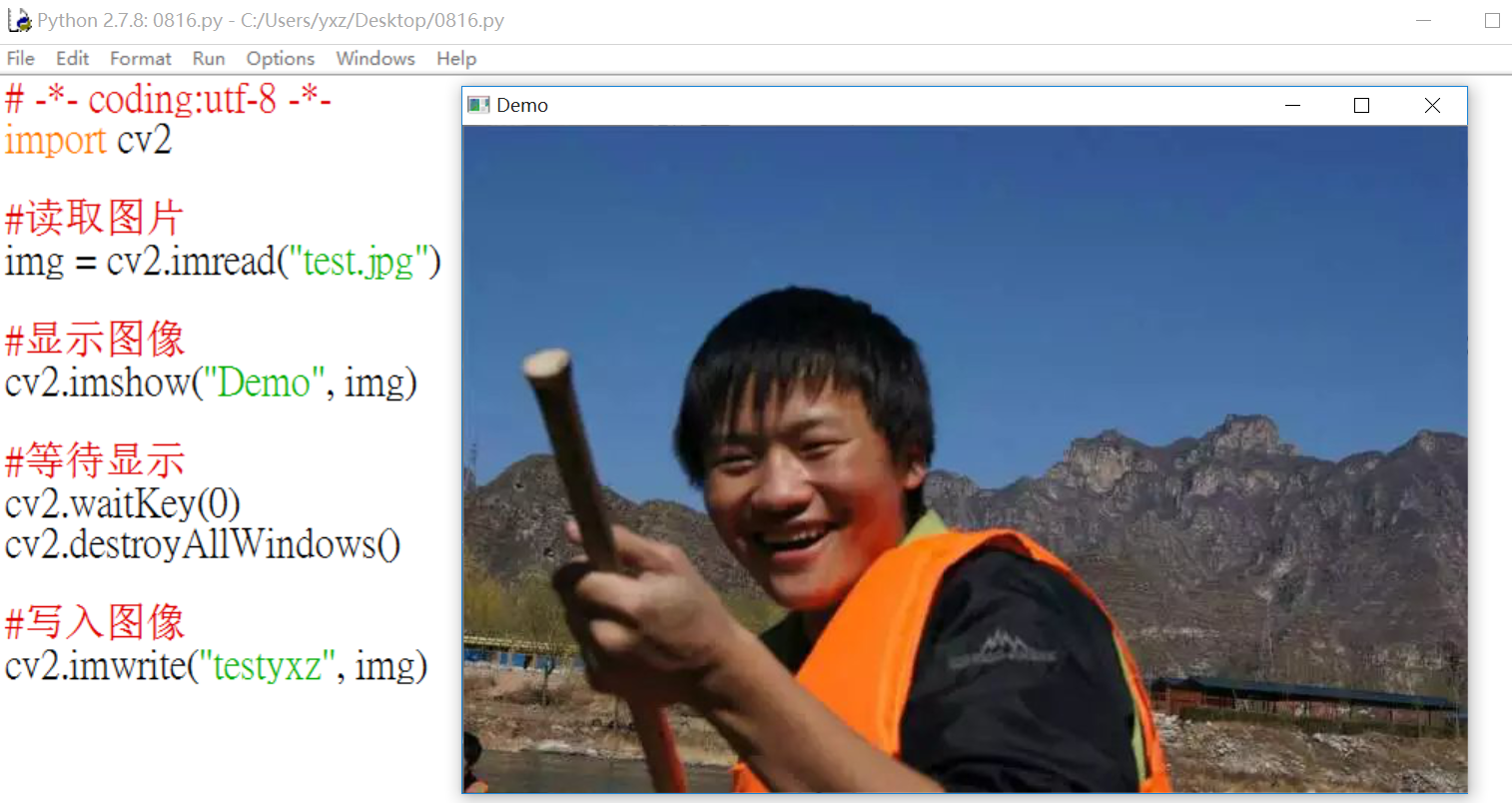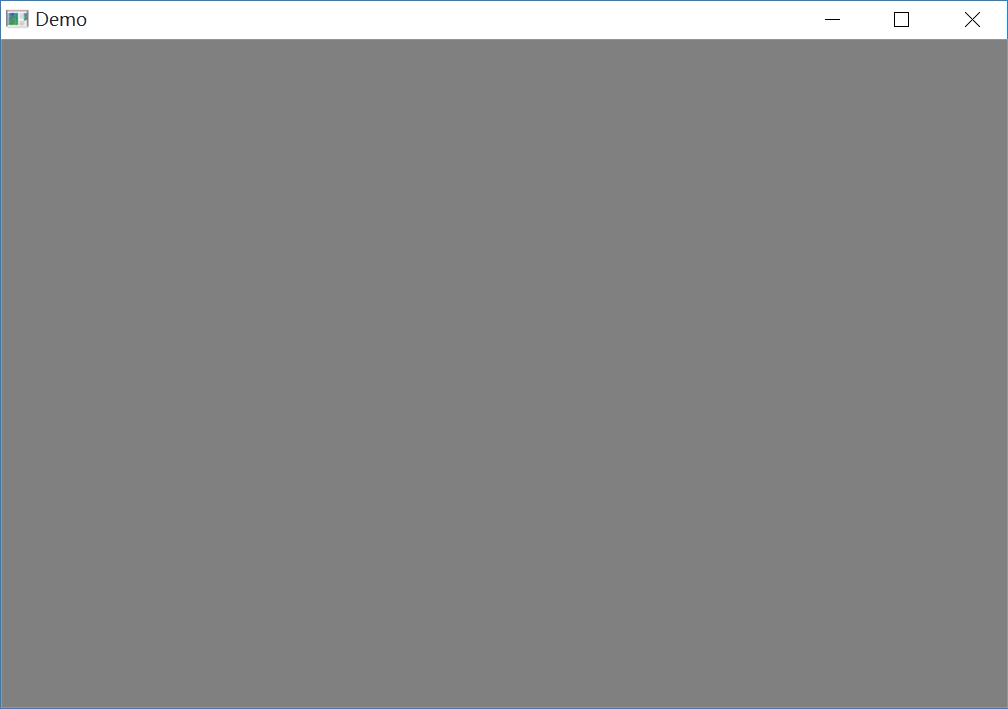``````#无限期等待输入
k=cv2.waitKey(0)
#如果输入ESC退出
if k==27:
cv2.destroyAllWindows()
``````

# 三.OpenCV像素处理

1.读取像素

eg: test=img[88,42]

eg: blue=img[88,142,0] green=img[88,142,1] red=img[88,142,2]

2.修改图像

img[88,142] = 255

img[88,142, 0] = 255
img[88,142, 1] = 255
img[88,142, 2] = 255

img[88,142] = [255, 255, 255]

``````# -*- coding:utf-8 -*-
import cv2

#读取图片
test = img[88,142]
print test
img[88,142] = [255, 255, 255]
print test

#分别获取BGR通道像素
blue = img[88,142,0]
print blue
green = img[88,142,1]
print green
red = img[88,142,2]
print red

#显示图像
cv2.imshow("Demo", img)

#等待显示
cv2.waitKey(0)
cv2.destroyAllWindows()

#写入图像
cv2.imwrite("testyxz.jpg", img)
``````

[158 107 64]
[255 255 255]
255
255
255

``````# -*- coding:utf-8 -*-
import cv2

#读取图片

#该区域设置为白色
img[100:200, 150:250] = [255,255,255]

#显示图像
cv2.imshow("Demo", img)

#等待显示
cv2.waitKey(0)
cv2.destroyAllWindows()

#写入图像
cv2.imwrite("testyxz.jpg", img)
``````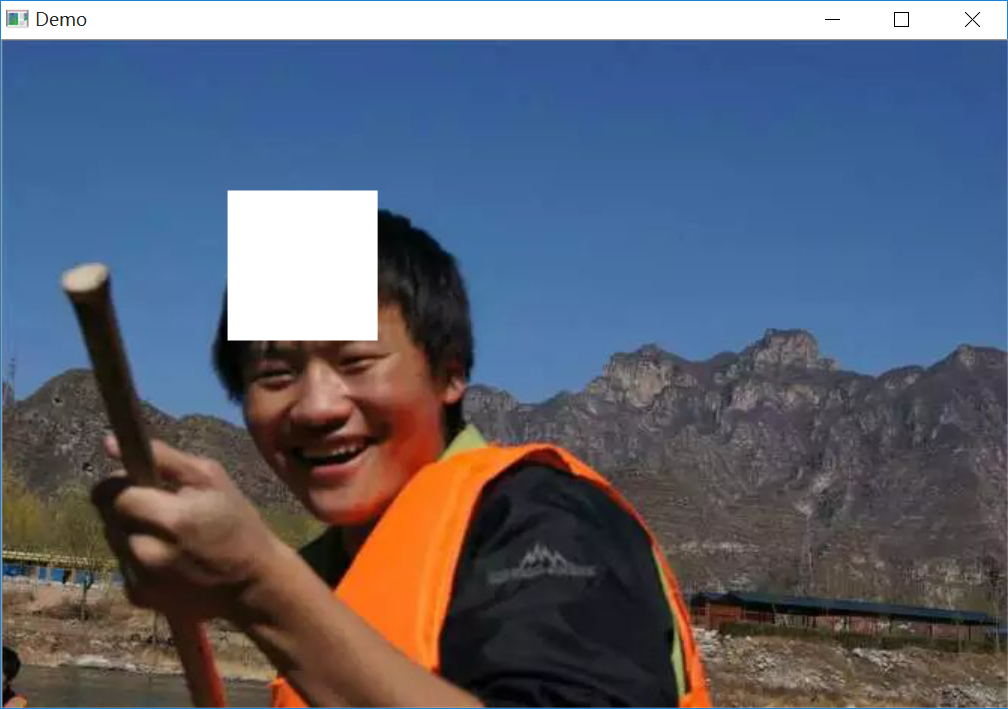（By：Eastmount 2018-08-16 夜11点 https://blog.csdn.net/Eastmount/

2016-01-01 12:29:14 lz20120808 阅读数 323
• ###### 区块链技术详解

区块链技术入门教程，该课程对目前区块链知识进行一次体系化的讲解，使得初学者能迅速抓住区块链技术的核心思想，对区块链应用和智能合约有一个的、由浅入深的了解。

12379 人正在学习 去看看 CSDN讲师

2015由研一走到研二，由老老实实上课到步入实验室接手项目。
本命年转眼即逝，不得不总结下自己的所做作为。

## 书籍阅读

《傲慢与偏见》，《愤怒的葡萄》，《少有人走的路》，《三体2》，《三十六谋略全书》、《这个国家会好吗:中国崛起的经济学分析》

《愤怒的葡萄》短评

## 《这个国家会好吗:中国崛起的经济学分析》

1. 利益分配中的破坏性要素：”以很低的成本给对方造成很大的伤害，从而从对方应该获得的报酬中拿走一部分报酬，即低成本伤害权，会造成破坏性要素参与分配”。所以政府要提高资本家的破坏成本。

1. 通货膨胀有外生性原因，也有内生性原因，外生性原因就是政府向市场投放更多的货币，而内生性原因实际上是金融杠杆。通货膨胀不仅对经济发展“没有用”，只能影响价格水平而不能提高实际产量，还对经济发展“有害”，会导致严重的经济资源的配置失衡和浪费。
2. 关于信仰。东西文化的差别不在于讲不讲为人处事，真正差别在于:东方认为人活在世界上最重要的就是为人处事，为人就是履行人生义务，处世就是协调个人生活与社会生活的关系。而西方文化则认为，除了为人处事。人生还有更重要的事情要做，敬仰上帝。所以东西方文化的根本差异不在于谁讲什么谁没讲什么而在于排列的顺序。

## 论文阅读

1. 查阅了视觉感知，图像插值相关论文。
2. 学习了相机标定的相关论文，从上半年接触相关论文，断断续续一直到下半年才有个整体认识。并在本博客做了下总结。理论联系实践才会有深刻理解，opencv提供了很大帮助。
3. 学习了图像拼接，相关的理论发展已经比较成熟，但是应用到项目中，还有很多注意点。
4. 翻译了一篇论文，（中译英），结果老师很不满意。甚是惭愧，一直想切实提高英语能力，但是没有坚持下来，只是靠背英语单词是不行的，要多看相关方向英文论文。

## 项目

1. 接手本实验室的项目。涉及标识符识别与缺陷检测的项目。做了很多的实现与调试。
2. 研究图像拼接相关内容。
3. 比较高兴地是用了两个月时间对android有了简单入门，并做了几个小的APP。 虽然参赛没获得成绩，也是有很大收获。

专业虽然非计算机，但是以后却要从事计算机相关工作，也就是名字高大上的攻城狮或者受鄙视的程序猿。因此编程能力是最重要的。自己一直在努力提高。
经常在网上碰到一些牛人的经历，因此感觉自己努力了这么多年，依然那么的平凡，渺小，所有的成绩都微不足道，路漫漫其修远兮，吾将上下而求索。